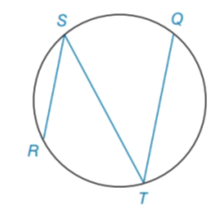Chapter 6.2, Problem 22E### Elementary Geometry for College St...

6th Edition
Daniel C. Alexander + 1 other
ISBN: 9781285195698

#### Solutions

Chapter
Section### Elementary Geometry for College St...

6th Edition
Daniel C. Alexander + 1 other
ISBN: 9781285195698
Textbook Problem
1 views

# In Exercises 21 and 22, complete each proof. Given: R S ¯ ∥ T Q ¯ Prove: R T ⌢ ≅ S Q ⌢PROOF Statements Reasons 1. R S ¯ ∥ T Q ¯ 1. ? 2. ∠ S ≅ ∠ T . 2. ? 3. ? . 3. If two ∠ s are ≅ , the ∠ s are = in measure. 4. m ∠ S = 1 2 m T R ⌢ . 4. ? 5. m ∠ T = 1 2 ( m S Q ⌢ ) 5. ? 6. 1 2 ( m R T ⌢ ) = 1 2 ( m S Q ⌢ ) 6. ? 7. m R T ⌢ = m S Q ⌢ 7. Multiplication Property ofEquality. 8. ? 8. If two arcs of a ⊙ are = in measure the arcs are ≅ .

To determine

To prove:

RTSQ.

Explanation

Given:

RS¯TQ¯

Proof:

 PROOF Statements Reasons 1. RS¯∥TQ¯ 1. Given 2. ∠S≅∠T. 2. Interior alternative angles are always equal. 3. m∠S=m∠T. 3. If two ∠s are ≅, the ∠s are = in measure. 4. m∠S=12(mRT⌢). 4. By theorem, the measure of an inscribed angle of a circle is half the measure of its intercept arc. 5. m∠T=12(mSQ⌢) 5

### Still sussing out bartleby?

Check out a sample textbook solution.

See a sample solution

#### The Solution to Your Study Problems

Bartleby provides explanations to thousands of textbook problems written by our experts, many with advanced degrees!

Get Started

#### In Exercises 914, evaluate the expression. 10. 5654

Applied Calculus for the Managerial, Life, and Social Sciences: A Brief Approach

#### True or False: is a convergent series.

Study Guide for Stewart's Single Variable Calculus: Early Transcendentals, 8th

#### What is the general purpose of a manipulation check?

Research Methods for the Behavioral Sciences (MindTap Course List)